## 2. 3. 2 Incidence and Traversal

In connection with the construction of a function space on a tesselation, it has to be determined, which cells cover an edge or a vertex or which vertices are covered by an edge. In general, it is not necessary to discriminate which of the sets is the subset and which is the superset but one only defines the property of incidencebetween two elements of which one is a subset of the other.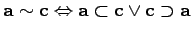(2.20)

Once the property of incidence is defined, one aims to find all elements which are incident with an initial element. Such a formulation is obtained, if the local support of a basis function is required. As an example, the local support of a shape function which is collocated with an edge point, is the set of all cells which are incident with the edge on which the point is located. Therefore, the following definition of such sets of incident elements turns out to be fruitful.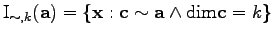(2.21)

Traversal is defined within a data structural context, because the members of the set of incident sets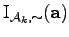have to be iterated or traversed, i.e. the set is examined element by element. Figure 2.4 shows all possible methods of incidence traversal for a three-dimensional cell complex consisting of tetrahedra.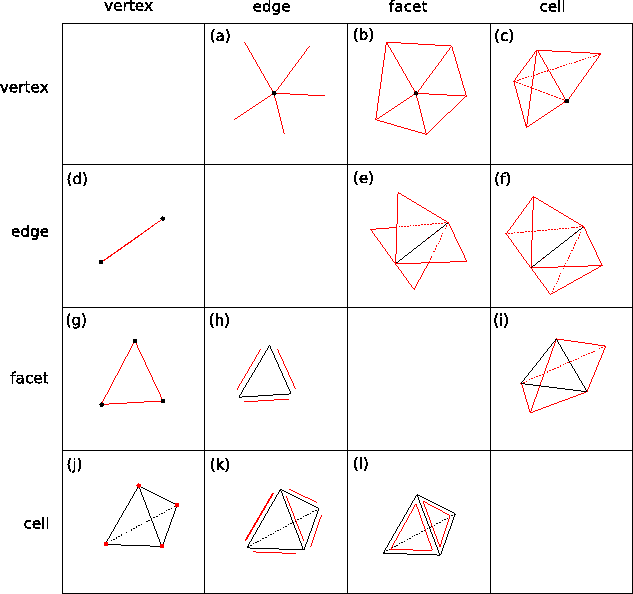In the following the traversal functions are given names consisting of two capital letters where the first letter denotes the basis element (V, E, F, C) and the second letter denotes the traversed element. For instance, the function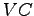stands for a function which returns all cells incident to the vertex passed. Additionally, traversal functions may have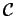andas first letter, wheredenotes the cell complex anddenotes the union of boundary facets of the respective cell complex. The following identities can be established between the incidence traversal function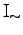and the specialized incidence traversal functions: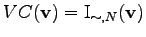(2.22)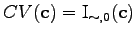(2.23)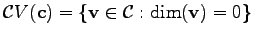(2.24)

Michael 2008-01-16